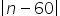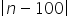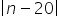# CAT-2021-QA-Slot-1/Free CAT Question papers

5).

The number of integers n that satisfy the inequality<<is

1. 21

2. 18

3. 20

4. 19

### Explanation

The inequality<holds good if n < 80.

The inequality<holds good if n > 60.

Combining both n < 80 and n > 60

Thus, n may take values from 61 to 79, i.e., 19 integers.

Ans (4)